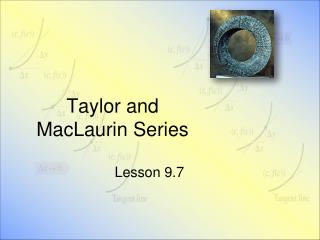DownloadDownload PresentationTaylor and MacLaurin Series

# Taylor and MacLaurin Series

Télécharger la présentation## Taylor and MacLaurin Series

- - - - - - - - - - - - - - - - - - - - - - - - - - - E N D - - - - - - - - - - - - - - - - - - - - - - - - - - -
##### Presentation Transcript

1. Taylor and MacLaurin Series Lesson 9.7

2. Centered at c or expanded about c Taylor & Maclaurin Polynomials • Consider a function f(x) that can be differentiated n times on some interval I • Our goal: find a polynomial function M(x) • which approximates f • at a number c in its domain • Initial requirements • M(c) = f(c) • M '(c) = f '(c)

3. Linear Approximations • The tangent line is a good approximation of f(x) for x near a True value f(x) Approx. value of f(x) f'(a) (x – a) (x – a) f(a) a x

4. Linear Approximations • Taylor polynomial degree 1 • Approximating f(x) for x near 0 • Consider • How close are these? • f(.05) • f(0.4) View Geogebra demo

5. Quadratic Approximations • For a more accurate approximation to f(x) = cos x for x near 0 • Use a quadratic function • We determine • At x = 0 we must have • The functions to agree • The first and second derivatives to agree

6. Quadratic Approximations • Since • We have

7. Quadratic Approximations • So • Now how close are these? View Geogebra demo

8. Taylor Polynomial Degree 2 • In general we find the approximation off(x) for x near 0 • Try for a different function • f(x) = sin(x) • Let x = 0.3

9. Higher Degree Taylor Polynomial • For approximating f(x) for x near 0 • Note for f(x) = sin x, Taylor Polynomial of degree 7 View Geogebra demo

10. Improved Approximating • We can choose some other value for x, say x = c • Then for f(x) = sin(x – c) the nth degree Taylor polynomial at x = c • Try for c =  / 3

11. Assignment • Lesson 9.7A • Page 658 • Exercises 1 – 4 all5 - 29 odd